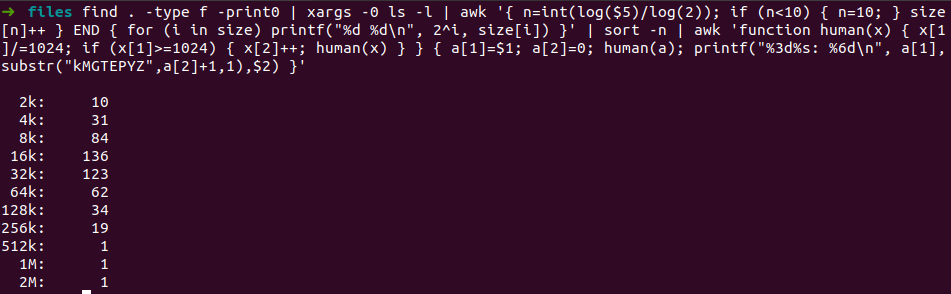# File size histogram via the command linefind . -type f -print0 | xargs -0 ls -l | awk '{ n=int(log($5)/log(2)); if (n<10) { n=10; } size[n]++ } END { for (i in size) printf("%d %d\n", 2^i, size[i]) }' | sort -n | awk 'function human(x) { x/=1024; if (x>=1024) { x++; human(x) } } { a=$1; a=0; human(a); printf("%3d%s: %6d\n", a,substr("kMGTEPYZ",a+1,1),\$2) }'